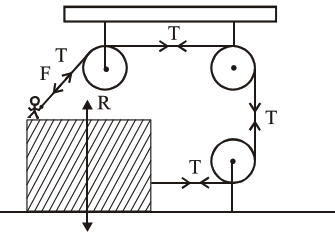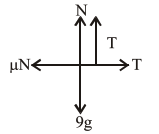# A boy of mass`
Question:

A boy of mass $4 \mathrm{~kg}$ is standing on a piece of wood having mass $5 \mathrm{~kg}$. If the coefficient of friction between the wood and the floor is $0.5$, the maximum force that the boy can exert on the rope so that the piece of wood does not move from its place is_________N.(Round off to the Nearest Integer)

[Take $\mathrm{g}=10 \mathrm{~ms}^{-2}$ ]Solution:$\mathrm{N}+\mathrm{T}=90$

$\mathrm{~T}=\mu \mathrm{N}=0.5(90-\mathrm{T})$

$1.5 \mathrm{~T}=45$

$\mathrm{T}=30$